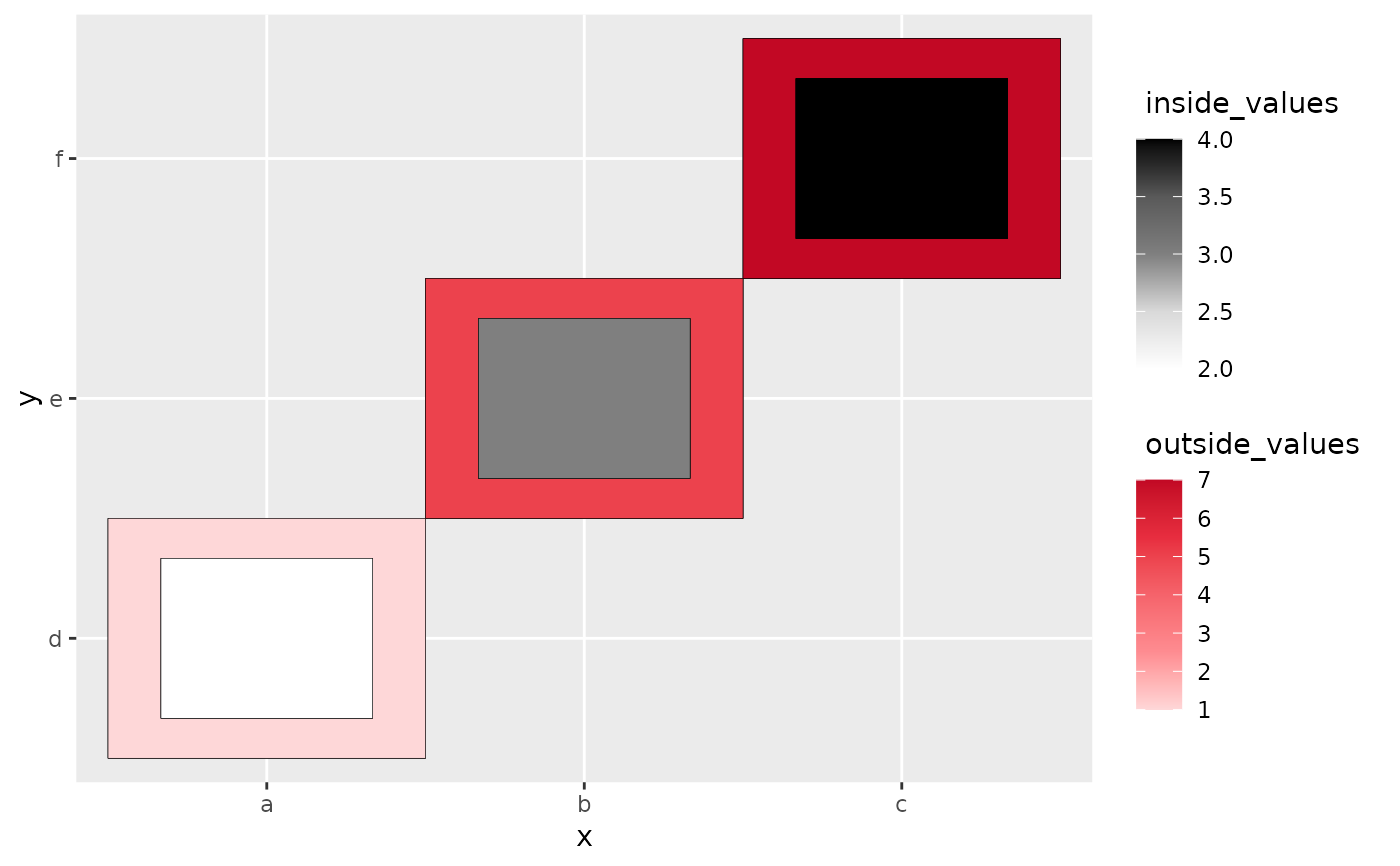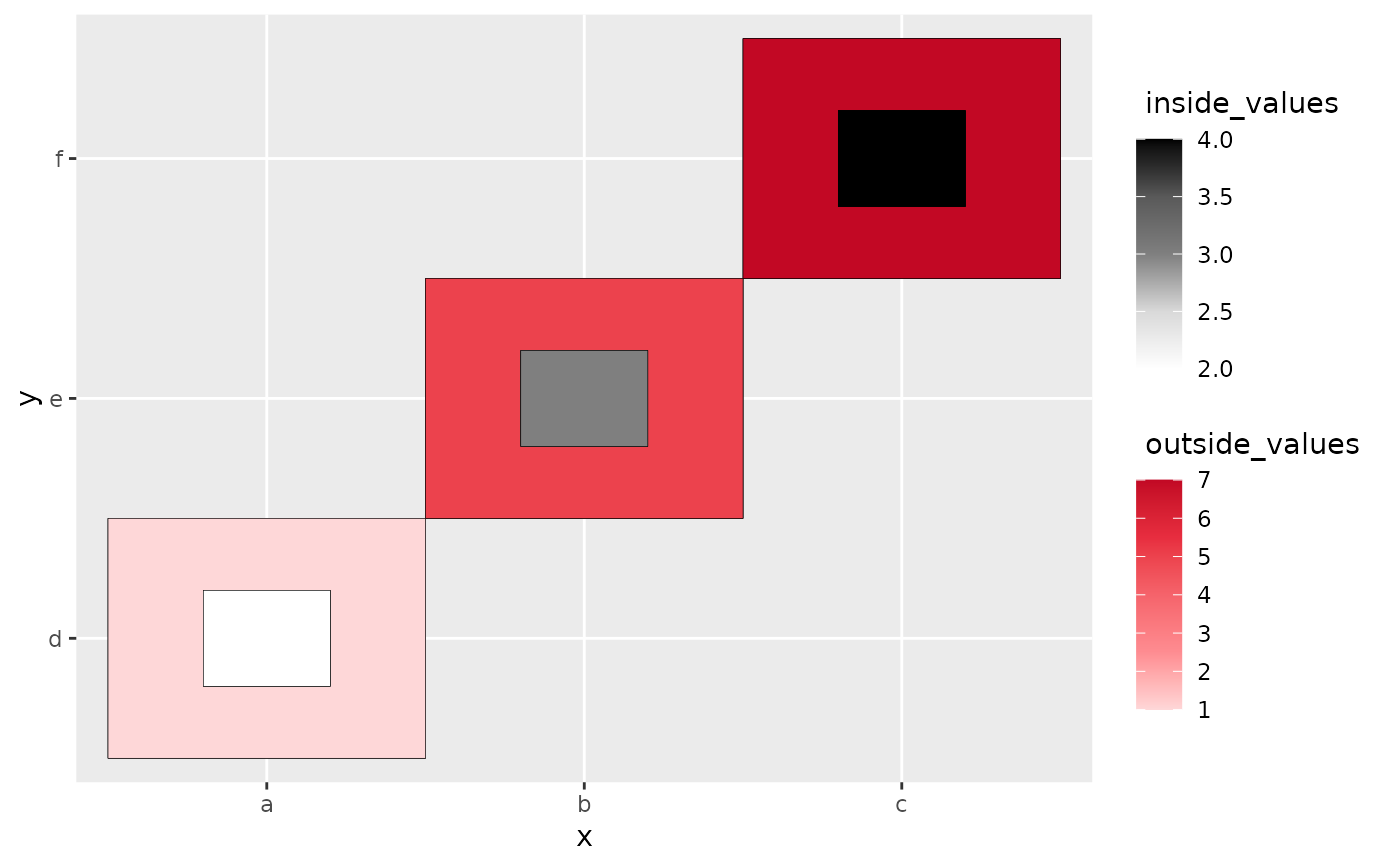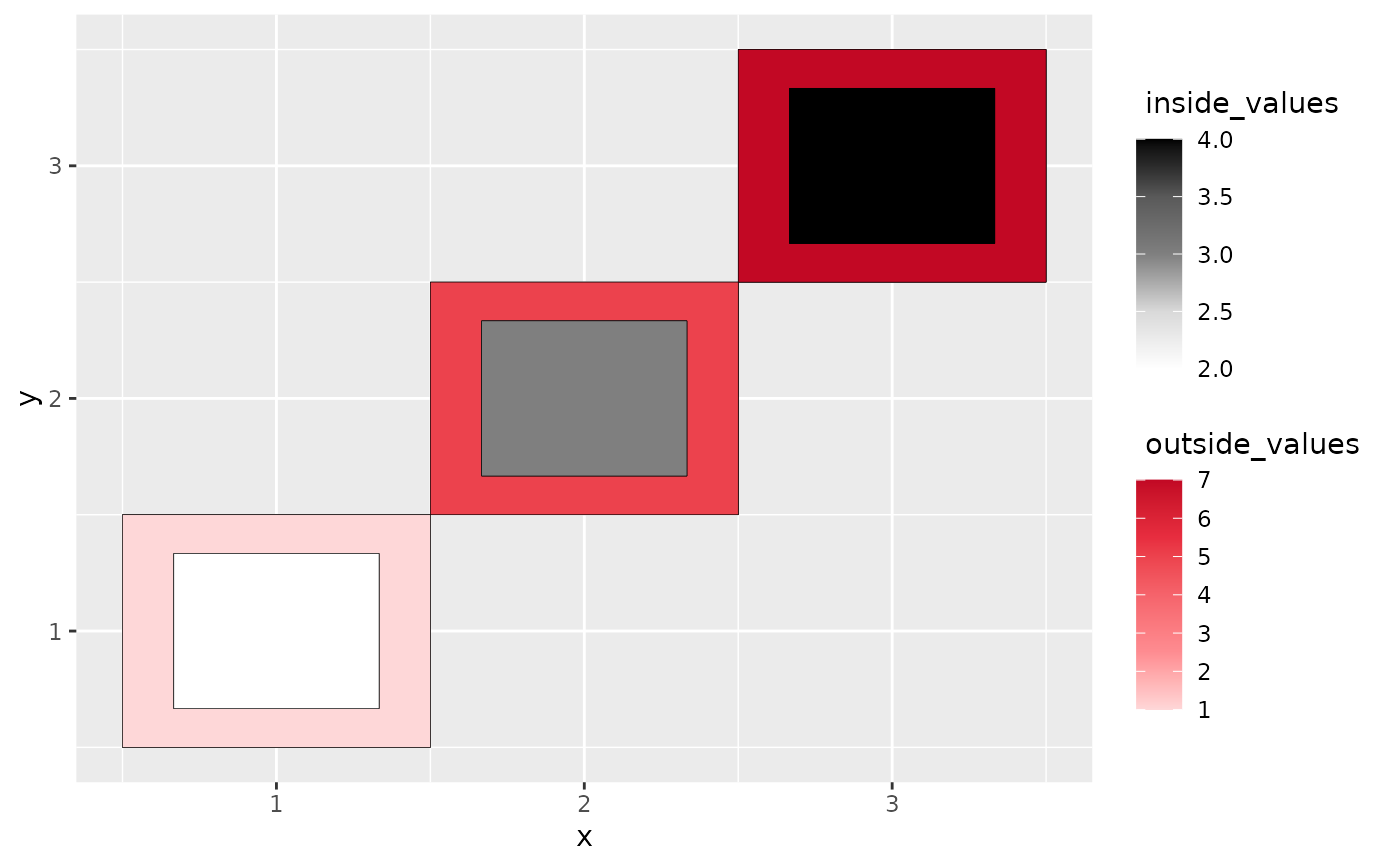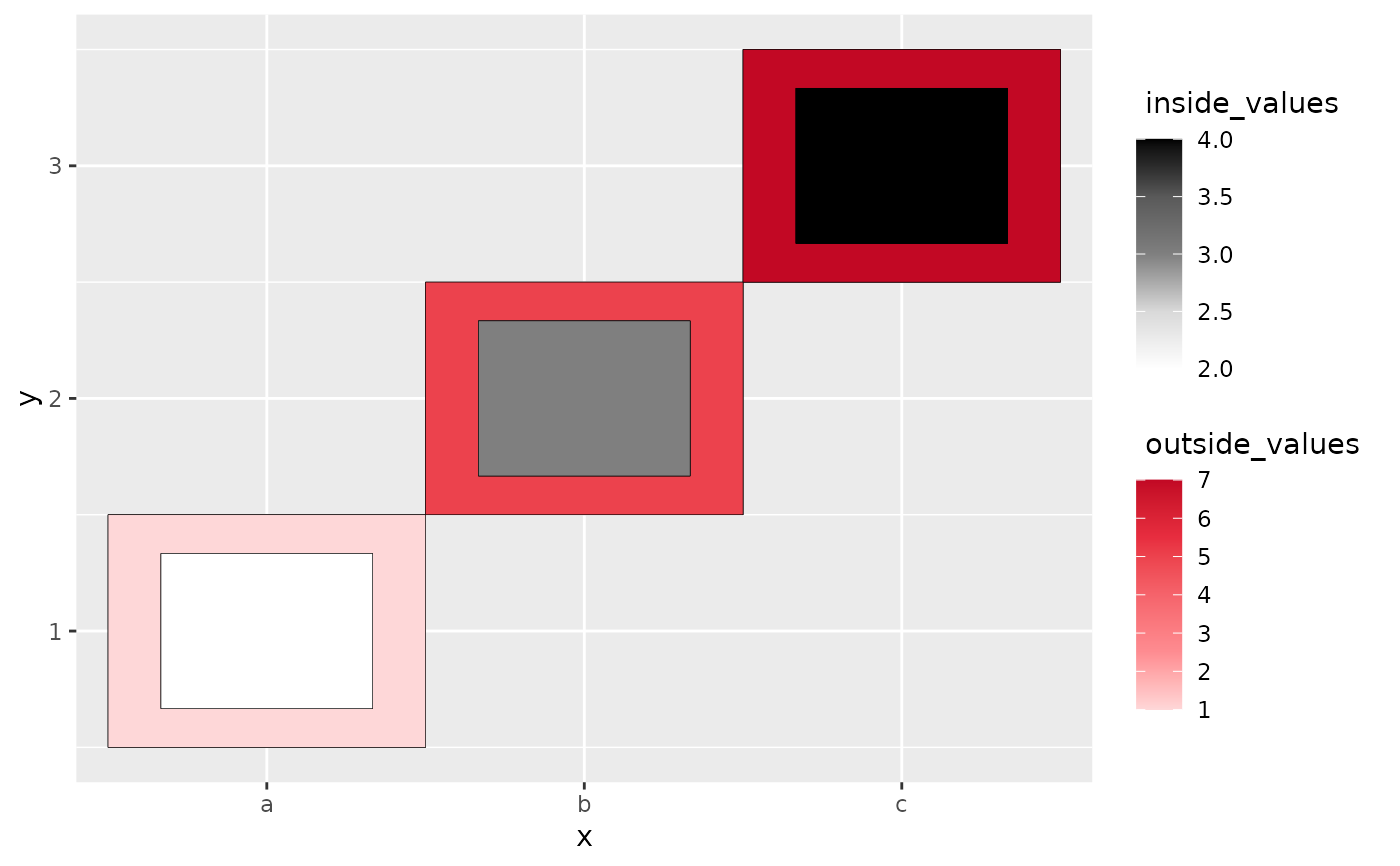The heatgrid geom is used to create a modified heat map that uses luminance to show the values from two sources on the same plot.

## Usage

geom_heat_grid(
outside,
outside_name = NULL,
outside_colors = c("#FED7D8", "#FE8C91", "#F5636B", "#E72D3F", "#C20824"),
inside,
inside_name = NULL,
inside_colors = c("gray100", "gray85", "gray50", "gray35", "gray0"),
r = 3,
...
)

## Arguments

outside

The column name for the outside portion of heatgrid.

outside_name

The label name (in quotes) for the legend of the outside rendering. Default is NULL.

outside_colors

A color vector, usually as hex codes.

inside

The column name for the inside portion of heatgrid.

inside_name

The label name (in quotes) for the legend of the inside rendering. Default is NULL.

inside_colors

A color vector, usually as hex codes.

r

The value that controls how large of the inside portion with respect to the outside one. When r is larger, the inside get smaller. Default value is 3.

...

... accepts any arguments scale_fill_gradientn() has .

## Value

A heatgrid comparing two data sources.

## Examples


# heatgrid with categorical variables only

library(ggplot2)

data <- data.frame(x = rep(c("a", "b", "c"), 3),
y = rep(c("d", "e", "f"), 3),
outside_values = rep(c(1,5,7),3),
inside_values = rep(c(2,3,4),3))

ggplot(data, aes(x,y)) +
geom_heat_grid(outside = outside_values,
inside = inside_values)# Making the inside smaller by setting r to be larger.

ggplot(data, aes(x,y)) +
geom_heat_grid(outside = outside_values,
inside = inside_values,
r = 5)# heatgrid with numeric variables only

data <- data.frame(x = rep(c(1, 2, 3), 3),
y = rep(c(1, 2, 3), 3),
outside_values = rep(c(1,5,7),3),
inside_values = rep(c(2,3,4),3))

ggplot(data, aes(x,y)) +
geom_heat_grid(outside = outside_values,
inside = inside_values)# heatgrid with a mixture of numeric and categorical variables

data <- data.frame(x = rep(c("a", "b", "c"), 3),
y = rep(c(1, 2, 3), 3),
outside_values = rep(c(1,5,7),3),
inside_values = rep(c(2,3,4),3))

ggplot(data, aes(x,y)) +
geom_heat_grid(outside = outside_values,
inside = inside_values)